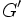# Three-step group

A finite group$G$ is said to be a three-step group if it satisfies all the following conditions:
• The commutator subgroup$G'$ has a permutable complement$Q$ which is a cyclic Hall subgroup
•$G'' \le F(G) \le G'$ where$F(G)$ is the Fitting subgroup of$G$
• If$H$ is a largest Hall subgroup of$G$ contained in$F(G)$, then$H$ is non-cyclic and$F(G) = HC_G(H)$
•$H$ contains a cyclic subgroup$R$ such that$C_{G'}(y) = R$ for all non-identity elements$y$ of$H$.